Example Questions

1 2 23 24 25 26 27 28 29 31 Next →

Example Question #8 : Transformation

The following is an equation of a circle: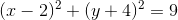If this circle is moved to the left 2 spaces and down 3 spaces, where does the center of the new circle lie?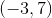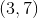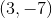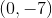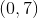Explanation:

The general formula for a circle with center (h,k) and radius r is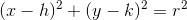.

The center of the original circle, therefore, is (2, -4).

If we move the circle to the left 2 spaces and down 3 spaces, then the center of the new circle is given by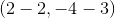orExample Question #9 : Transformation

Let f(x) = x3 – 2x2 + x +4. If g(x) is obtained by reflecting f(x) across the y-axis, then which of the following is equal to g(x)?

–x3 + 2x2 – x + 4

x3 + 2x2 + x + 4

x3 – 2x2 – x + 4

–x3 – 2x2 – x – 4

–x3 – 2x2 – x + 4

–x3 – 2x2 – x + 4

Explanation:

In order to reflect a function across the y-axis, all of the x-coordinates of every point on that function must be multiplied by negative one. However, the y-values of each point on the function will not change. Thus, we can represent the reflection of f(x) across the y-axis as f(-x). The figure below shows a generic function (not f(x) given in the problem) that has been reflected across the y-axis, in order to offer a better visual understanding.Therefore, g(x) = f(–x).

f(x) = x3 – 2x2 + x – 4

g(x) = f(–x) = (–x)3 – 2(–x)2 + (–x) + 4

g(x) = (–1)3x3 –2(–1)2x2 – x + 4

g(x) = –x3 –2x2 –x + 4.

The answer is –x3 –2x2 –x + 4.

Example Question #10 : Transformation

Bobby draws a circle on graph paper with a center at (2, 5) and a radius of 10.

Jenny moves Bobby's circle up 2 units and to the right 1 unit.

What is the equation of Jenny's circle?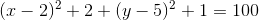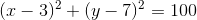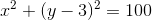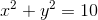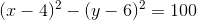Explanation:

If Jenny moves Bobby's circle up 2 units and to the right 1 unit, then the center of her circle is (3, 7). The radius remains 10.

The general equation for a circle with center (h, k) and radius r is given byFor Jenny's circle, (h, k) = (3, 7) and r=10.

Substituting these values into the general equation gives usExample Question #5 : How To Find A Ray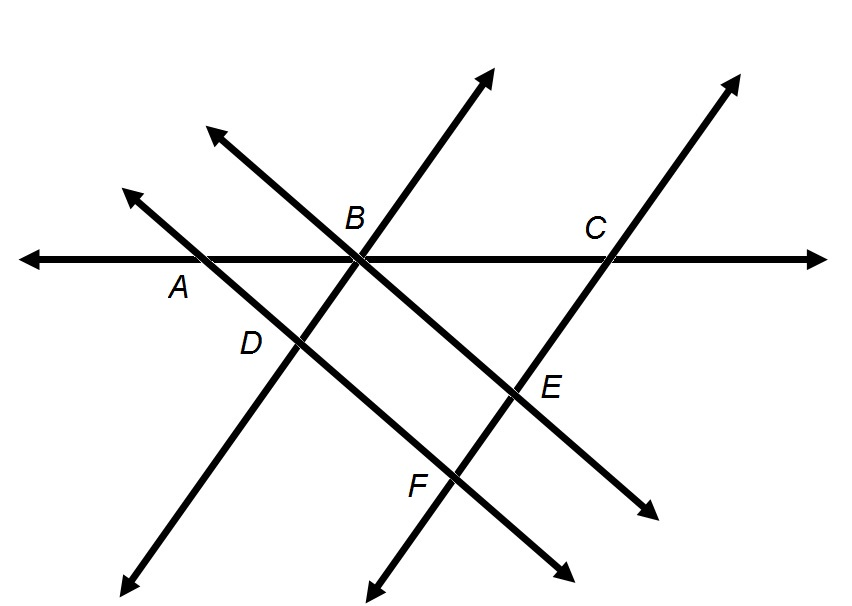Refer to the above diagram. The plane containing the above figure can be called Plane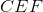.

True

False

False

Explanation:

A plane can be named after any three points on the plane that are not on the same line. As seen below, points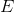,  andare on the same line.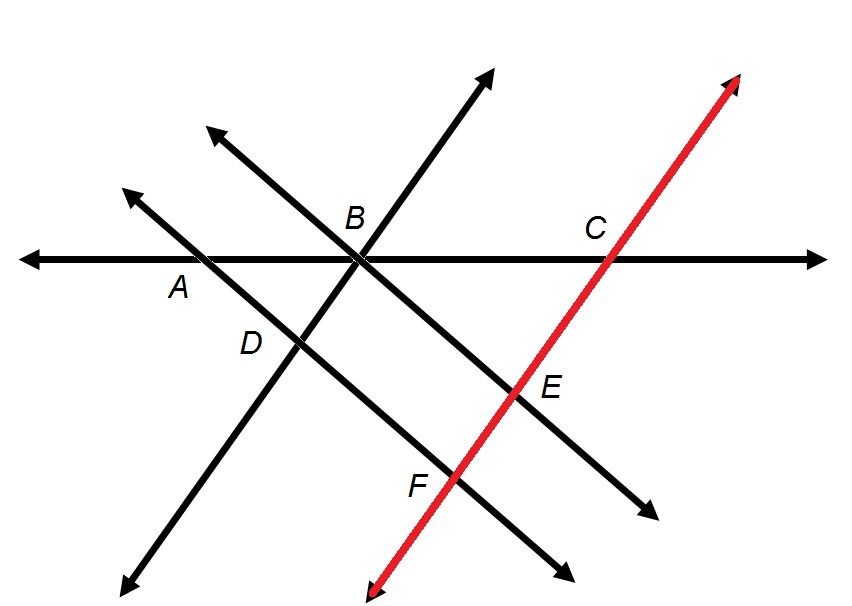Therefore, Planeis not a valid name for the plane.

Example Question #1543 : Basic GeometryRefer to the above figure.

True or false: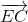and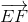comprise a pair of opposite rays.

False

True

True

Explanation:

Two rays are opposite rays, by definition, if

(1) they have the same endpoint, and

(2) their union is a line.

The first letter in the name of a ray refers to its endpoint; the second refers to the name of any other point on the ray.andboth have endpoint, so the first criterion is met.passes through pointandpasses through pointandare indicated below in green and red, respectively: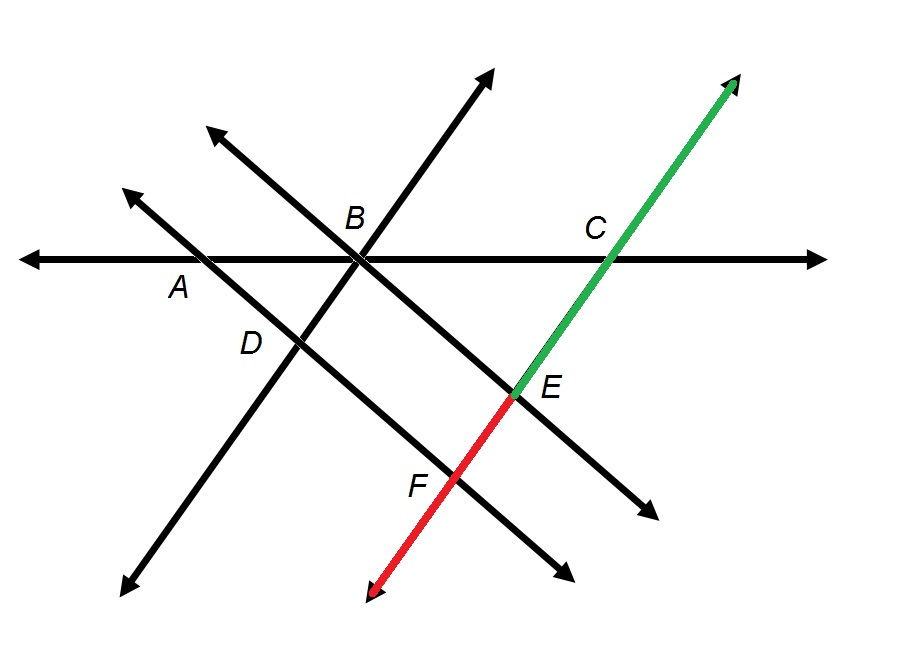The union of the two rays is a line. Both criteria are met, so the rays are indeed opposite.

Example Question #1548 : Basic GeometryRefer to the above diagram:

True or false: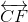may also called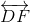.

True

False

False

Explanation:

A line can be named after any two points it passes through. The lineis indicated in green below.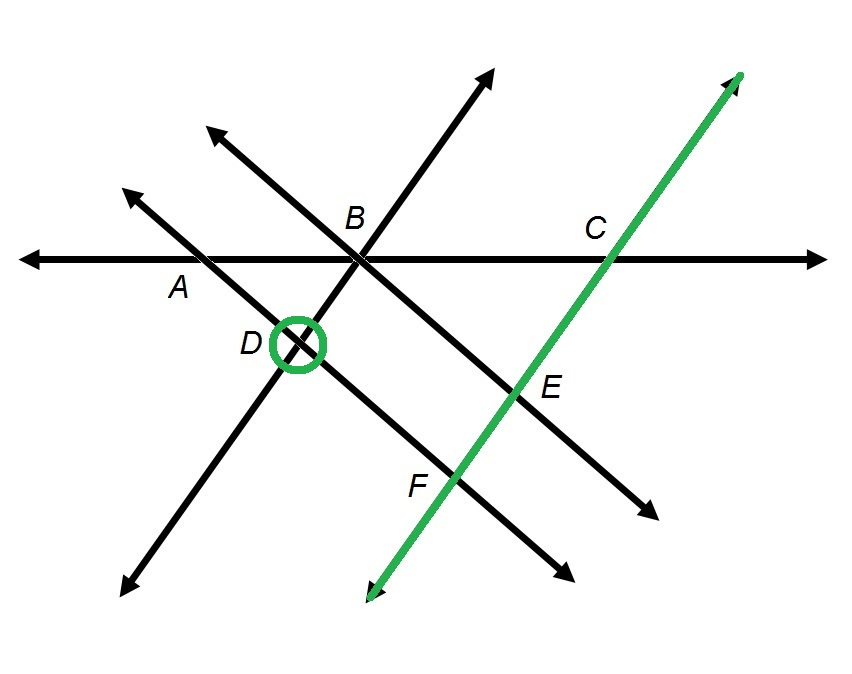The line does not pass through, socannot be part of the name of the line. Specifically,is not a valid name.

Example Question #301 : Coordinate Geometry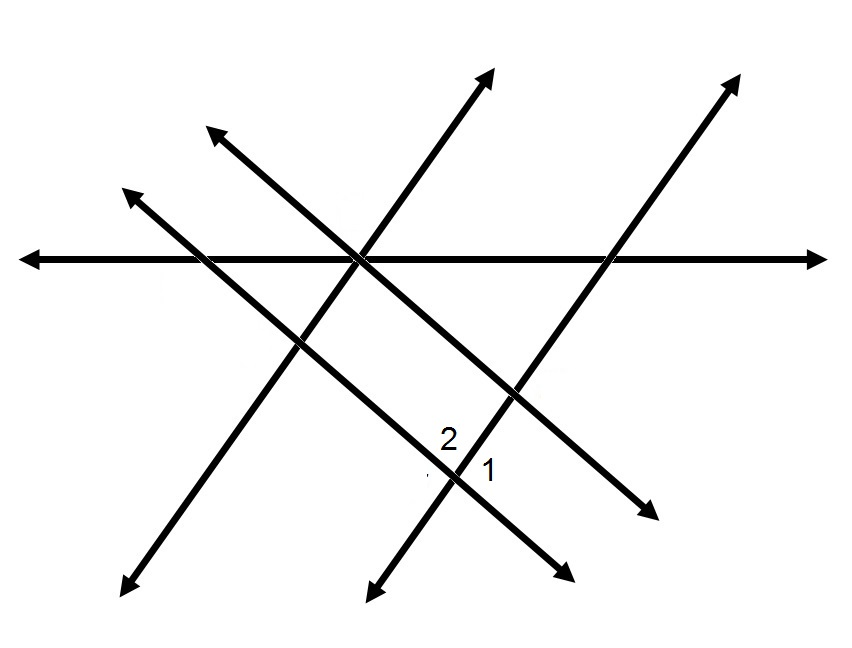Refer to the above diagram.

True or false: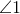and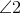comprise a pair of vertical angles.

False

True

False

Explanation:

By definition, two angles comprise a pair of vertical angles if

(1) they have the same vertex; and

(2) the union of the two angles is exactly a pair of intersecting lines.

In the figure below,andare marked in green and red, respectively: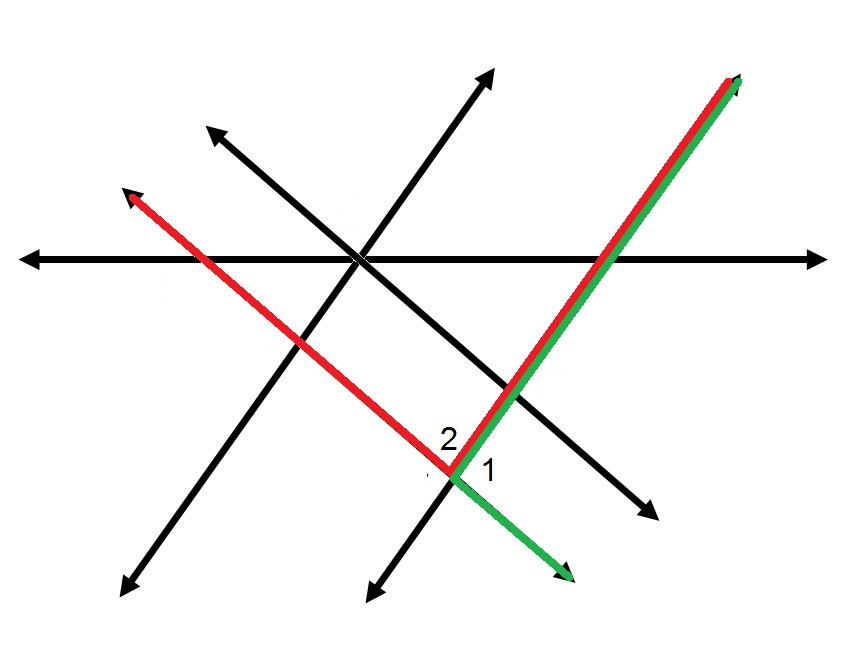While the two angles have the same vertex, their union is not a pair of intersecting lines. The two angles are not a vertical pair.

Example Question #42 : How To Find An Angle Of A Line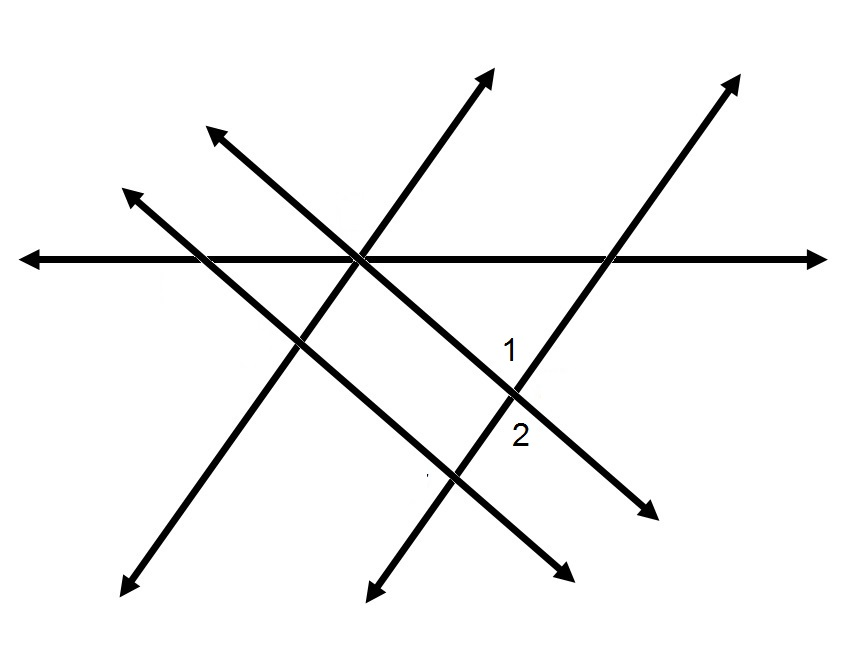Refer to the above diagram.

True or false:andcomprise a linear pair.

False

True

False

Explanation:

By definition, two angles form a linear pair if and only if

(1) they have the same vertex;

(2) they share a side; and,

(3) their interiors have no points in common.

In the figure below,andare marked in green and red, respectively: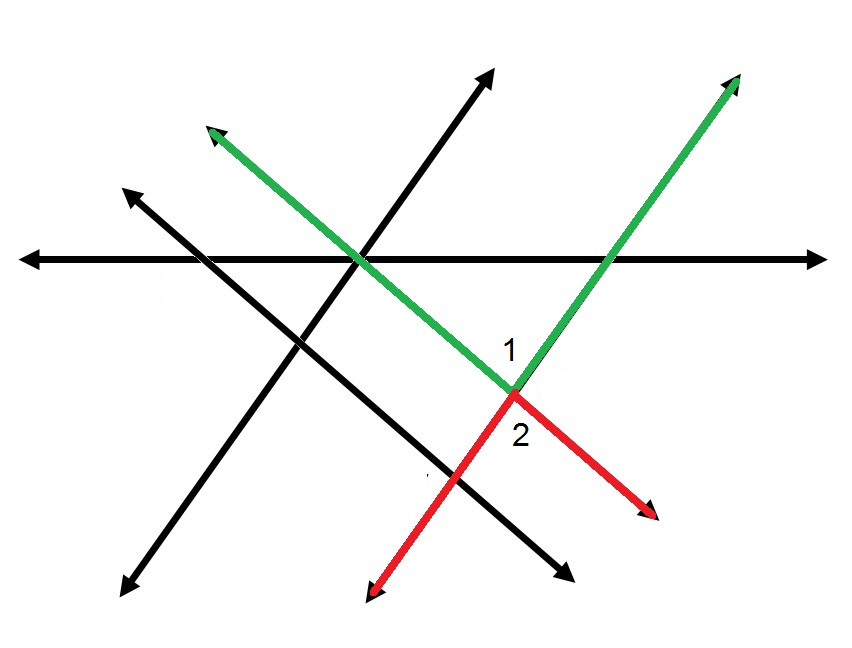The two angles have the same vertex and share no interior points. However, they do not share a side. Therefore, they do not comprise a linear pair.

1 2 23 24 25 26 27 28 29 31 Next →